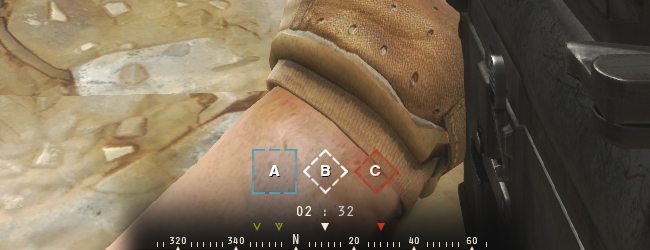Log InMaking a direction compassmorningtoastDoes anyone have an example of a horizontal directional compass in a game?

The type of compass that is just a strip and moves left/right as the character rotates on screen? So like facing .25 in rotation would be North and so on from there. I don't need waypoints indicated, just have the direction be in the middle when the character is facing that direction.

Example:I'm working to figure it out as we speak but this time I remembered to poll the community before I do a bunch of work, lol.

I think my struggle is how to turn the rotation of the character in speed for the horizontal compass and so the compass letters line up when a cardinal direction is hit.

Any ideas to approach or pointers, tips, examples are appreciated.

P#113187 2022-06-15 14:37 ( Edited 2022-06-15 14:50)Here's code that almost recreates your visual. I'm not sure how to convince pico-8 to not clip text at the left edge though, and that seems to be preventing "W" from showing when looking directly north. Still, this might be helpful. (assuming you didn't already figure it out yourself).

The key is that pico-8's quirk of using 0-1 as the range for sin and cos means that the direction can be directly used as the fraction of the length to shift the bar. From there you just need to know that the total length of the bar would be twice what's shown on screen if you want the two perpendicular directions to show too.

The reason I used 230 for the total length with an offset to how it's shown is so that the letters would be more readable. 256 would be more accurate if the bar is actually the entire bottom of the screen.

 ```function _init() dir = 0 end function _update() if btn(⬅️) then dir += .01 end if btn(➡️) then dir -= .01 end while dir < 0 do dir+=1end while dir > 1 do dir-=1end end function _draw() cls() color(6) circ(64,64,10) color(8) line(64,64,64+15*cos(dir),64+15*sin(dir)) color(9) local edir = dir +.25 if edir >= 1 then edir -=1 end local sdir = dir +.5 if sdir >= 1 then sdir -=1 end local wdir = dir +.75 if wdir >= 1 then wdir -=1 end print("n",230*dir + 8,120) print("e",230*edir + 8,120) print("s",230*sdir + 8,120) print("w",230*wdir + 8,120) end```
P#113191 2022-06-15 15:39Awesome, thanks for sharing. I was heading down a very similar path but just trying different values based on the specific speed I had chosen. Yours lets the rotation speed change and everything else syncs up nicely.

I think this will work great - thank you so much! I'll see what I can do with this, should be useful in my game.

P#113194 2022-06-15 17:42@kimiyoribaka - Your code worked great! I was able to transpose it a bit into the following (~150 tokens). I'm going to connect this into my game and see how it feels. There's a HUD UI already so I hope the compass doesn't make it too cluttered.

 ```-- Math for X position of compass letters function compass_x(v) local d=compass_dir+v if d>=1 then d-=1 end return compass_w*d+compass_off end -- MAIN LOOP function _init() -- initial values; default direction, width of strip in pixels, offset for strip compass_dir,compass_w,compass_off=0,256,0 end function _update() if btn(0) then compass_dir+=.008 end if btn(1) then compass_dir-=.008 end while compass_dir<0 do compass_dir+=1 end while compass_dir>1 do compass_dir-=1 end end function _draw() cls(1) -- rotation display; for demo only circ(64,64,10,7) line(64,64,64+15*cos(compass_dir),64+15*sin(compass_dir),8) print(compass_dir,50,2,7) -- compass strip; cardinal directions print("n",compass_x(0),120,7) print("e",compass_x(.25),120,7) print("s",compass_x(.5),120,7) print("w",compass_x(.75),120,7) -- optional intercardinal directions print("NE",compass_x(.125),120,6) print("SE",compass_x(.375),120,6) print("SW",compass_x(.625),120,6) print("NW",compass_x(.875),120,6) end```
P#113197 2022-06-15 18:38 ( Edited 2022-06-15 18:38)